## Electron Self Energy

Even in classical electromagnetism, if one can calculates the energy needed to assemble an electron, the result is infinite, yet electrons exist. The quantum self energy correction is also infinite although it can be rendered finite if we accept the fact that out theories are not valid up to infinite energies.

The quantum self energy correction has important, measurable effects. It causes observable energy shifts in Hydrogen and it helps us solve the problem of infinities due to energy denominators from intermediate states.

The coupled differential equations from first order perturbation theory for the state under study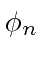and intermediate states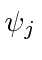may be solved for the self energy correction.The result is, in general, complex. The imaginary part of the self energy correction is directly related to the width of the state.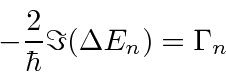The time dependence of the wavefunction for the state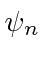is modified by the self energy correction.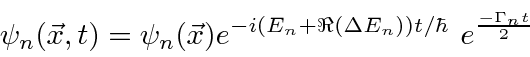This gives us the exponential decay behavior that we expect, keeping resonant scattering cross sections from going to infinity.

The real part of the correction should be studied to understand relative energy shifts of states. It is the difference between the bound electron's self energy and that for a free electron in which we are interested. The self energy correction for a free particle can be computed.We automatically account for this correction by a change in the observed mass of the electron. For the non-relativistic definition of the energy of a free electron, an increase in the mass decreases the energy.If we cut off the integral at, the correction to the mass is only about 0.3%,

Since the observed mass of the electron already accounts for most of the self energy correction for a bound state, we must correct for this effect to avoid double counting of the correction. The self energy correction for a bound state then is.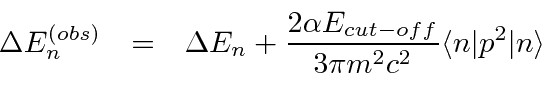In 1947, Willis E. Lamb and R. C. Retherford used microwave techniques to determine the splitting between theandstates in Hydrogen. The result can be well accounted for by the self energy correction, at least when relativistic quantum mechanics is used. Our non-relativistic calculation gives a qualitative explanation of the effect.Jim Branson 2013-04-22